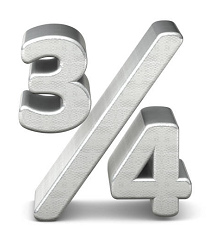# Fraction Calculator

Now you can Add Fractions, Subtract Fractions, Multiply Fractions, or Divide Fractions online. Find your answer in the simplest form with this Online Fraction Calculator.

First Fraction /
(+, -, x, ÷)
Second Fraction /

Subtract Fractions
Multiply Fractions
Divide Fractions

To reduce a fraction to the simplest form, try our Simplify Fractions Calculator

## How do you add fractions?

There are two cases regarding the denominators when we add ordinary fractions that are shown as follow in steps:

### Adding fractions that have like denominators:

• Simply add the numerators of the fractions.
• The denominator of the resulting fraction will be the common denominator of the fractions.
• Reduce the resulting fraction.
For example: a/b + d/b = (a + d)/b
or
7/17 + 9/17 = (7 + 9)/17

### Adding fractions with different denominators:

• If the denominators don’t match, Multiply the denominators together.
• Adjust your numerators (top numbers) accordingly. E.g. if you doubled the denominator, then double its numerator.
• Add together the numerators, and put this total over the common denominator.
• Simplify the fraction to the smallest possible denominator, with the numerators also reduced proportionately.
Example: a/b + c/d = (ad + cb)/bd
For adding the fractions 1/3 and 1/5,
1/3 + 1/5 = (1*5 + 1*3)/3*5 = 5+3/15 = 8/15
The sum 8/15 is already in its simplest form.

## How do you subract fractions?

There are two cases regarding the denominators when we subtract ordinary fractions that are shown as follow with steps:

### Subtracting fractions with like denominators:

• Simply subtract the numerators of the fractions.
• The denominator of the resulting fraction will be the common denominator of the fractions.
• Reduce the resulting fraction.
An example
• a/d - c/d = (a-c)/d
• 4/18 - 3/18 = ( 4 - 3)/18 = 1/18

### Subtracting fractions with different denominators:

• If the denominators don’t match Multiply the denominators together.
• Adjust your numerators (top numbers) accordingly. E.g. if you doubled the denominator, then double its numerator.
• subtract the numerators, and put this total over the common denominator.
• Simplify the fraction to the smallest possible denominator, with the numerator also reduced proportionately.
a/b - c/d = (ad - cb)/bd
For subtracting the fractions 1/3 and 1/5,
1/3 - 1/5 = (1*5 - 1*3)/3*5 = 5-3/15 = 2/15
Example: For subtracting the fractions 10/15 and 1/5,
10/15 - 1/5 = (10*5 - 1*15)/15*5 = 50-15/75 = 35/75 = 7/15

## How do you multiply fractions?

Multiplying fractions is fairly straightforward. Unlike adding and subtracting, it is not necessary to compute a common denominator in order to multiply fractions, multiplying fractions is shown as follow in steps:

• Simply, the numerators and denominators of each fraction are multiplied.
• The result forms a new numerator and denominator.
• If possible, the solution should be simplified. Refer to the equations below for clarification.
a/b × c/d = a*c / b*d
Example: Multiply the fractions 10/15 and 1/5.
10/15 × 1/5 =10/75
or =2/15

## How do you divide fractions?

For dividing the fractions the steps are as follows:
• You first find the reciprocal of the second fraction.
• Then multiply both fractions to get the result.
Example:
For dividing the fraction a/b and c/d the reciprocal of c/d is d/c.
You then multiply the first fraction by the reciprocal of the second fraction.
a/b ÷ c/d =a/b x d/c = a*d / b*c
Example 2: For dividing the fractions 1/5 and 15/4.
1/5 ÷ 15/4 = 1/5 x 4/15 = 4/75
The answer is 4/75. This answer is already in its simplest form.